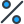# Taylor surveys students in one grade level who own at least one pet. She finds that 50% of the students surveyed own 2 pets, 3 students own 3 pets each, and 2 students own 4 pets each. Eight of the students in the grade own 1 pet. Considering the number of pets as the random variable, X, which of the following is the probability distribution, PX(x)? A probability distribution is shown. The probability of 1 is 0.3; 2 is 0.5; 3 is 0.11; 4 is 0.08. A probability distribution is shown. The probability of 1 is 0.28; 2 is 0.5; 3 is 0.1; 4 is 0.13. A probability distribution is shown. The probability of 1 is 0.61; 2 is 0.5; 3 is 0.22; 4 is 0.15. A probability distribution is shown. The probability of 1 is 0.53; 2 is 0.5; 3 is 0.2; 4 is 0.27.

•Subject:

Mathematics
• Author:

• Created:

7 months ago

Step-by-step explanation:

We know that half of the students has two pets. The rest of the students make up the other half. So, we have 3 students + 2 students + 8 students = 13 students that make half of the sample population

That means total number of students being surveyed is 13+13=26 students

Then we work out the probability

P(One pet) = 8/26 = 4/13

P(Two pets) = 1/2

P(Three pets) = 3/26

P( Four pets) = 2/26 = 1/13

The probability distribution is shown in the table below. Let  be the number of pets and  is the probability of owning the number of pets

First, we need to work out the total number of students who were being surveyed.

We know that half of the students has two pets. The rest of the students make up the other half. So, we have 3 students + 2 students + 8 students = 13 students that make half of the sample population

That means total number of students being surveyed is 13+13=26 students

Then we work out the probability

P(One pet) = 8/26 = 4/13

P(Two pets) = 1/2

P(Three pets) = 3/26

P( Four pets) = 2/26 = 1/13

The probability distribution is shown in the table below. Let  be the number of pets and  is the probability of owning the number of pets

:)

Don't worry! There are several alternative approaches you can try to resolve your query. Here are some tips to help you find answers in different ways:

1. Reframe your question: Sometimes, the way you phrase your question can limit your search results. Try rephrasing it using different keywords or providing more context to get better results.
2. Utilize social media: Post your question on social media platforms, particularly those focused on professional or specialized topics. Twitter, LinkedIn, and Facebook groups can connect you with individuals who may have relevant expertise or experiences to share.
3. Consult subject matter experts: Reach out to experts in the field related to your question. Many professionals are willing to help and share their knowledge. You can connect with them through email, LinkedIn messages, or by attending relevant conferences or events.
4. Use our website to find your question through the search box above, or you can sign up to ask your question for our big educational community. Our experts will review your question, and you will get a quick and quality answer.
5. Collaborate with others: If your question is related to a specific project or problem, consider collaborating with others who might have complementary skills or knowledge. Teamwork can lead to innovative solutions and shared insights.

Remember, the process of finding answers often involves persistence, creativity, and an open mind. By exploring various resources, reaching out to others, and being proactive in your search, you increase your chances of finding the information you need. Happy quest for knowledge!